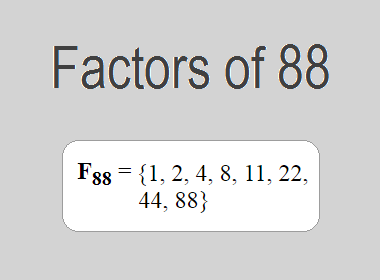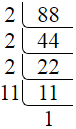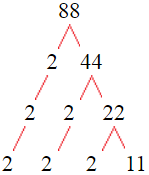# Factors of 88The factors of 88 are 1, 2, 4, 8, 11, 22, 44, and 88 i.e. F88 = {1, 2, 4, 8, 11, 22, 44, 88}. The factors of 88 are all the numbers that can divide 88 without leaving a remainder.

We can check if these numbers are factors of 88 by dividing 88 by each of them. If the result is a whole number, then the number is a factor of 88. Let's do this for each of the numbers listed above:

·        1 is a factor of 88 because 88 divided by 1 is 88.

·        2 is a factor of 88 because 88 divided by 2 is 44.

·        4 is a factor of 88 because 88 divided by 4 is 22.

·        8 is a factor of 88 because 88 divided by 8 is 11.

·        11 is a factor of 88 because 88 divided by 11 is 8.

·        22 is a factor of 88 because 88 divided by 22 is 4.

·        44 is a factor of 88 because 88 divided by 44 is 2.

·        88 is a factor of 88 because 88 divided by 88 is 1.

## How to Find Factors of 88?

1 and the number itself are the factors of every number. So, 1 and 88 are two factors of 88. To find the other factors of 88, we can start by dividing 88 by the numbers between 1 and 88. If we divide 88 by 2, we get a remainder of 0. Therefore, 2 is a factor of 88. If we divide 88 by 3, we get a remainder of 1. Therefore, 3 is not a factor of 88.

Next, we can check if 4 is a factor of 88. If we divide 88 by 4, we get a remainder of 0. Therefore, 4 is also a factor of 88. We can continue this process for all the possible factors of 88.

Through this process, we can find that the factors of 88 are 1, 2, 4, 8, 11, 22, 44, and 88. These are the only numbers that can divide 88 without leaving a remainder.

********************

********************

## Properties of the Factors of 88

The factors of 88 have some interesting properties. One of the properties is that the sum of the factors of 88 is equal to 180. We can see this by adding all the factors of 88 together:

1 + 2 + 4 + 8 + 11 + 22 + 44 + 88 = 180

Another property of the factors of 88 is that the prime factors of 88 are 2, and 11 only.

## Applications of the Factors of 88

The factors of 88 have several applications in mathematics. One of the applications is in finding the highest common factor (HCF) of two or more numbers. The HCF is the largest factor that two or more numbers have in common. For example, to find the HCF of 88 and 55, we need to find the factors of both numbers and identify the largest factor they have in common. The factors of 88 are 1, 2, 4, 8, 11, 22, 44, and 88. The factors of 55 are 1, 5, 11, and 55. The largest factor that they have in common is 11. Therefore, the HCF of 88 and 55 is 11.

Another application of the factors of 88 is in prime factorization. Prime factorization is the process of expressing a number as the product of its prime factors. The prime factors of 88 are 2, and 11 since these are the only prime numbers that can divide 88 without leaving a remainder. Therefore, we can express 88 as:

88 = 2 × 2 × 2 × 11

We can do prime factorization by division and factor tree method also. Here is the prime factorization of 88 by division method,88 = 2 × 2 × 2 × 11

Here is the prime factorization of 88 by the factor tree method,88 = 2 × 2 × 2 × 11

## Conclusion

The factors of 88 are the numbers that can divide 88 without leaving a remainder. The factors of 88 are 1, 2, 4, 8, 11, 22, 44, and 88. The factors of 88 have some interesting properties, such as having a sum of 180. The factors of 88 have several applications in mathematics, such as finding the highest common factor and prime factorization.